﻿ 普遍n口网络的等效电压源定理 A General n-Port Network’s Equivalent Voltage Source Theorem

Open Journal of Circuits and Systems
Vol.05 No.02(2016), Article ID:17513,7 pages
10.12677/OJCS.2016.52003

A General n-Port Network’s Equivalent Voltage Source Theorem

Runsheng Liang

Wuhan University of Science and Technology, Wuhan Hubei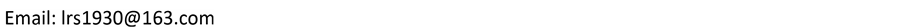Received: Apr. 16th, 2016; accepted: May 7th, 2016; published: May 10th, 2016ABSTRACT

This paper presents a general theorem for an n-port linear time-invariant network contained several sources can be expressed by equivalent voltage sources which equal to the open port voltages and a passive network which is the original network when its contained sources don’t work. Obviously, the conventional Thevenin’s theorem is only a special case of it for n = 1  .

Keywords:Time-Invariant, Equivalent Voltage-Source, Open-Port Voltage, Impedance Matrix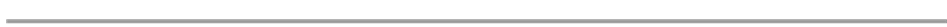1. 前言

2. 推导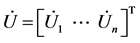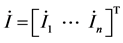Figure 1. NS connects NLFigure 2. NS disconnects NL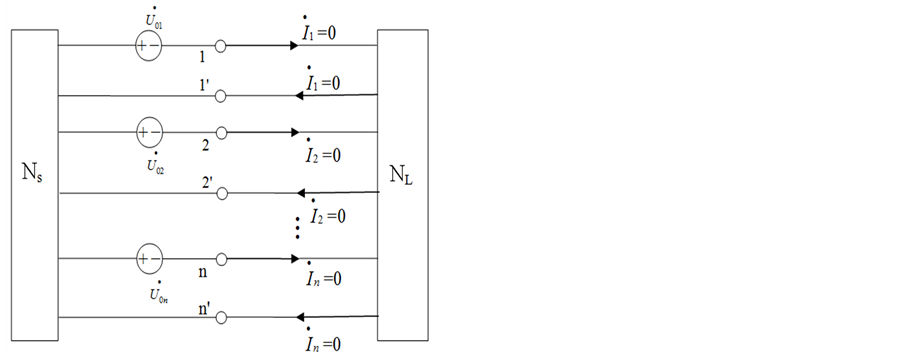Figure 3. Offseting the effect of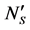source by connecting a voltage source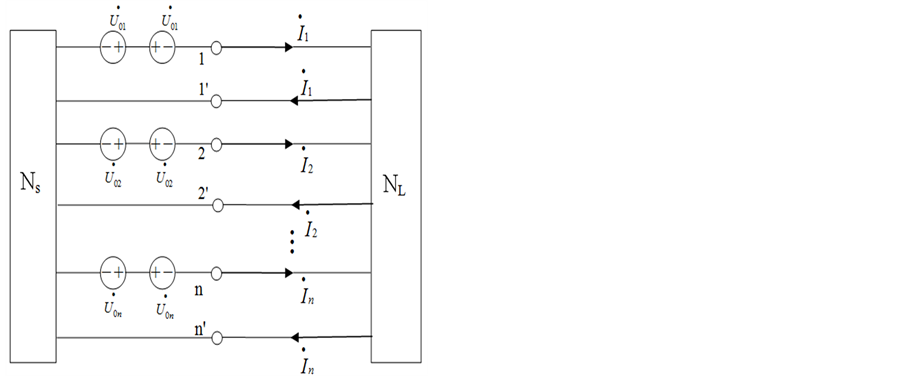Figure 4. Two series voltage sources cancel out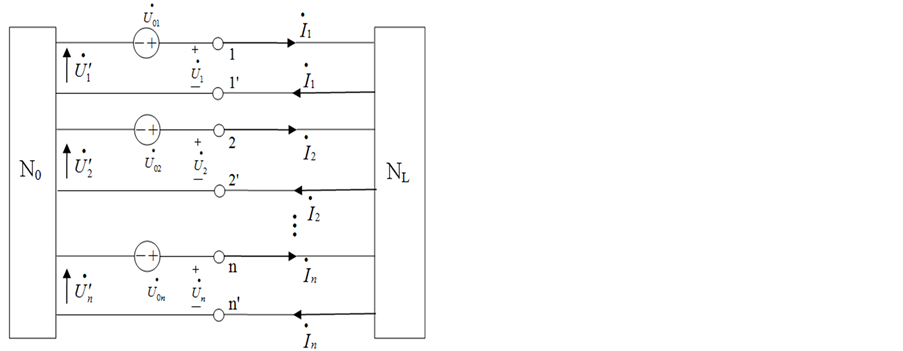Figure 5. The 2nd series voltage source acts at N0, NL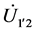，第二次再由相量三角形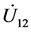上已定出，可定出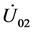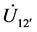，第三次由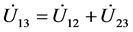上已定出，故可定出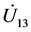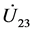，第四次由上已定出。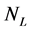为线性时不变无源连通网络,它的端口电压与端口电流是线性关系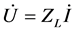(1)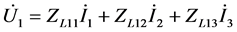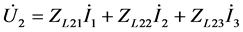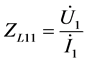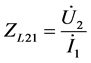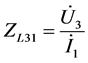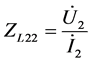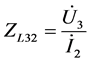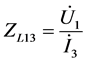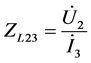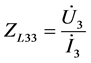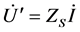(2)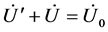(3)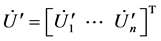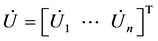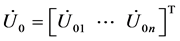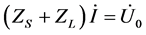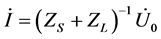(4)

3. 特例A General n-Port Network’s Equivalent Voltage Source Theorem[J]. 电路与系统, 2016, 05(02): 21-27. http://dx.doi.org/10.12677/OJCS.2016.52003

1. 1. 俞大光. 电工基础(上册) [M]. 北京: 人民教育出版社, 1964: 205.

Chen and Liang, A General n-Port Network Reciprocity Theorem (IEEE 1990).